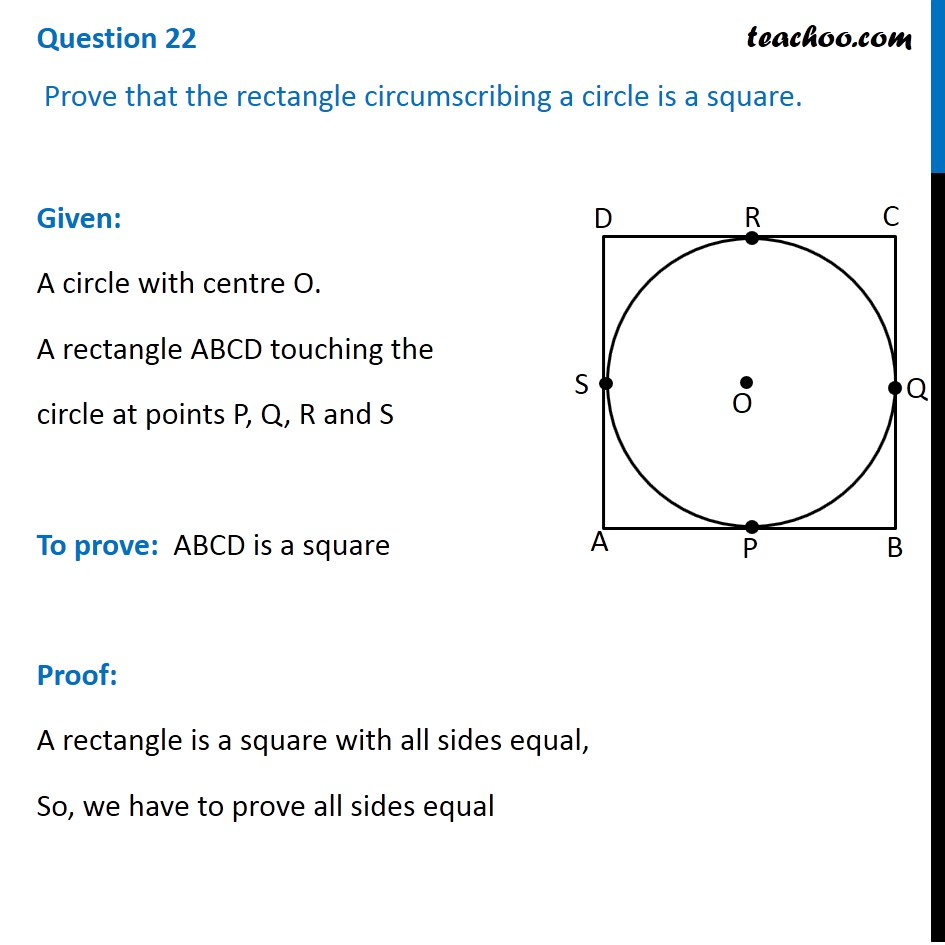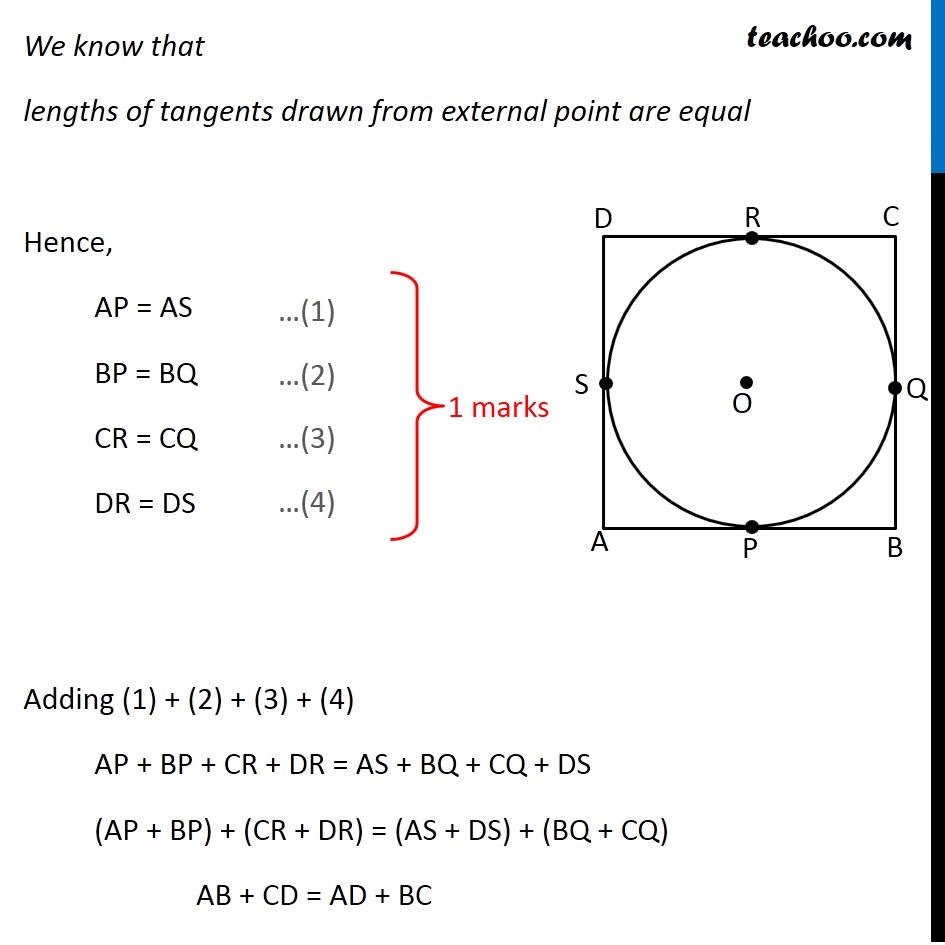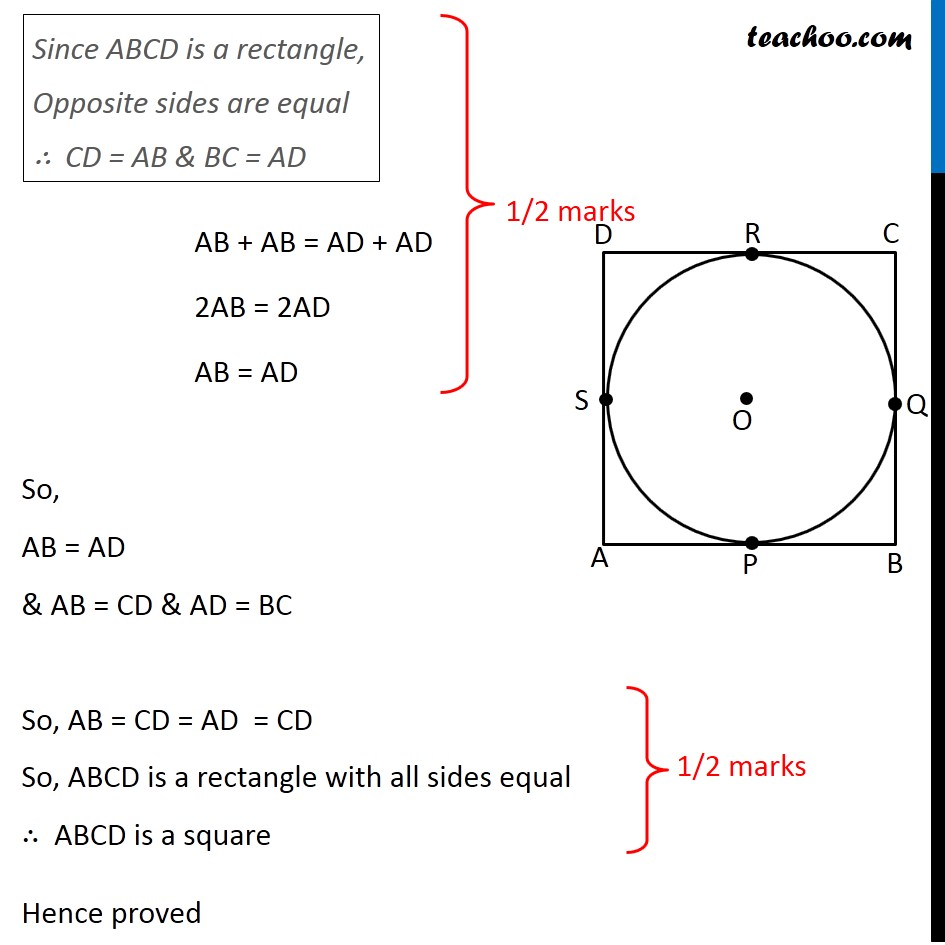CBSE Class 10 Sample Paper for 2020 Boards - Maths Standard

Class 10
Solutions of Sample Papers for Class 10 Boards

## Prove that the rectangle circumscribing a circle is a square.Note : This is similar to Ex 10.2, 11 of NCERT – Chapter 10 Class 10

Learn in your speed, with individual attention - Teachoo Maths 1-on-1 Class

### Transcript

Question 22 Prove that the rectangle circumscribing a circle is a square. Given: A circle with centre O. A rectangle ABCD touching the circle at points P, Q, R and S To prove: ABCD is a square Proof: A rectangle is a square with all sides equal, So, we have to prove all sides equal We know that lengths of tangents drawn from external point are equal Hence, AP = AS BP = BQ CR = CQ DR = DS Adding (1) + (2) + (3) + (4) AP + BP + CR + DR = AS + BQ + CQ + DS (AP + BP) + (CR + DR) = (AS + DS) + (BQ + CQ) AB + CD = AD + BC …(1) …(2) …(3) …(4) Since ABCD is a rectangle, Opposite sides are equal ∴ CD = AB & BC = AD AB + AB = AD + AD 2AB = 2AD AB = AD So, AB = AD & AB = CD & AD = BC So, AB = CD = AD = CD So, ABCD is a rectangle with all sides equal ∴ ABCD is a square Hence proved